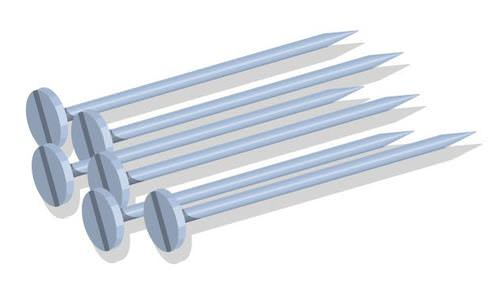# 12.3: Mass-Mole Stoichiometry

$$\newcommand{\vecs}{\overset { \rightharpoonup} {\mathbf{#1}} }$$ $$\newcommand{\vecd}{\overset{-\!-\!\rightharpoonup}{\vphantom{a}\smash {#1}}}$$$$\newcommand{\id}{\mathrm{id}}$$ $$\newcommand{\Span}{\mathrm{span}}$$ $$\newcommand{\kernel}{\mathrm{null}\,}$$ $$\newcommand{\range}{\mathrm{range}\,}$$ $$\newcommand{\RealPart}{\mathrm{Re}}$$ $$\newcommand{\ImaginaryPart}{\mathrm{Im}}$$ $$\newcommand{\Argument}{\mathrm{Arg}}$$ $$\newcommand{\norm}{\| #1 \|}$$ $$\newcommand{\inner}{\langle #1, #2 \rangle}$$ $$\newcommand{\Span}{\mathrm{span}}$$ $$\newcommand{\id}{\mathrm{id}}$$ $$\newcommand{\Span}{\mathrm{span}}$$ $$\newcommand{\kernel}{\mathrm{null}\,}$$ $$\newcommand{\range}{\mathrm{range}\,}$$ $$\newcommand{\RealPart}{\mathrm{Re}}$$ $$\newcommand{\ImaginaryPart}{\mathrm{Im}}$$ $$\newcommand{\Argument}{\mathrm{Arg}}$$ $$\newcommand{\norm}{\| #1 \|}$$ $$\newcommand{\inner}{\langle #1, #2 \rangle}$$ $$\newcommand{\Span}{\mathrm{span}}$$$$\newcommand{\AA}{\unicode[.8,0]{x212B}}$$Figure $$\PageIndex{1}$$ (Credit: CK-12 Foundation; Source: CK-12 Foundation; License: CK-12 Curriculum Materials license)

### Need nails?

When you are doing a large construction project, you have a good idea of how many nails you will need (lots!). When you go to the hardware store, you don’t want to sit there and count out several hundred nails. You can buy nails by weight, so you determine how many nails are in a pound, calculate how many pounds you need, and you’re on your way to begin building.

While the mole ratio is ever-present in all stoichiometry calculations, amounts of substances in the laboratory are most often measured by mass. Therefore, we need to use mole-mass calculations in combination with mole ratios to solve several different types of mass-based stoichiometry problems.

## Mass to Moles Problems

In this type of problem, the mass of one substance is given, usually in grams. From this, you are to determine the amount in moles of another substance that will either react with or be produced from the given substance.

$\text{mass of given} \rightarrow \text{moles of given} \rightarrow \text{moles of unknown}\nonumber$

The mass of the given substance is converted into moles by use of the molar mass of that substance from the periodic table. Then, the moles of the given substance are converted into moles of the unknown by using the mole ratio from the balanced chemical equation.

##### Example $$\PageIndex{1}$$: Mass-Mole Stoichiometry

Tin metal reacts with hydrogen fluoride to produce tin (II) fluoride and hydrogen gas, according to the following balanced equation.

$\ce{Sn} \left( s \right) + 2 \ce{HF} \left( g \right) \rightarrow \ce{SnF_2} \left( s \right) + \ce{H_2} \left( g \right)\nonumber$

How many moles of hydrogen fluoride are required to react completely with $$75.0 \: \text{g}$$ of tin?

###### Known
• Given: $$75.0 \: \text{g} \: \ce{Sn}$$
• Molar mass of $$\ce{Sn} = 118.69 \: \text{g/mol}$$
• $$1 \: \text{mol} \: \ce{Sn} = 2 \: \ce{mol} \: \ce{HF}$$ (mole ratio)
###### Unknown
• mol ​​​​​​​HF

Use the molar mass of $$\ce{Sn}$$ to convert the grams of $$\ce{Sn}$$ to moles. Then use the mole ratio to convert from $$\text{mol} \: \ce{Sn}$$ to $$\text{mol} \: \ce{HF}$$. This will be done in a single two-step calculation.

$$\text{g} \: \ce{Sn} \rightarrow \text{mol} \: \ce{Sm} \rightarrow \text{mol} \: \ce{HF}$$

Step 2: Solve.

$75.0 \: \text{g} \: \ce{Sn} \times \frac{1 \: \text{mol} \: \ce{Sn}}{118.69 \: \text{g} \: \ce{Sn}} \times \frac{2 \: \text{mol} \: \ce{HF}}{1 \: \text{mol} \: \ce{Sn}} = 1.26 \: \text{mol} \: \ce{HF}\nonumber$

###### Step 3: Think about your result.

The mass of tin is less than one mole, but the 1:2 ratio means that more than one mole of $$\ce{HF}$$ is required for the reaction. The answer has three significant figures because the given mass has three significant figures.

## Moles to Mass Problems

In this type of problem, the amount of one substance is given in moles. From this, you are to determine the mass of another substance that will either react with or be produced from the given substance.

$\text{moles of given} \rightarrow \text{moles of unknown} \rightarrow \text{mass of unknown}\nonumber$

The moles of the given substance are first converted into moles of the unknown by using the mole ratio from the balanced chemical equation. Then, the moles of the unknown are converted into mass in grams by use of the molar mass of that substance from the periodic table.

##### Example $$\PageIndex{2}$$: Mole-Mass Stoichiometry

Hydrogen sulfide gas burns in oxygen to produce sulfur dioxide and water vapor:

$2 \ce{H_2S} \left( g \right) + 3 \ce{O_2} \left( g \right) \rightarrow 2 \ce{SO_2} \left( g \right) + 2 \ce{H_2O} \left( g \right)\nonumber$

What mass of oxygen gas is consumed in a reaction that produces $$4.60 \: \text{mol} \: \ce{SO_2}$$?

###### Known
• Given: $$4.60 \: \text{mol} \: \ce{SO_2}$$
• $$2 \: \text{mol} \: \ce{SO_2} = 3 \: \text{mol} \: \ce{O_2}$$ (mole ratio)
• Molar mass of $$\ce{O_2} = 32.00 \: \text{g/mol}$$
###### Unknown
• mass O2 = ? g

Use the mole ratio to convert from $$\text{mol} \: \ce{SO_2}$$ to $$\text{mol} \: \ce{O_2}$$. Then convert $$\text{mol} \: \ce{O_2}$$ to grams. This will be done in a single two-step calculation.

$$\text{mol} \: \ce{SO_2} \rightarrow \text{mol} \: \ce{O_2} \rightarrow \text{g} \: \ce{O_2}$$

###### Step 2: Solve.

$4.60 \: \text{mol} \: \ce{SO_2} \times \frac{3 \: \text{mol} \: \ce{O_2}}{2 \: \text{mol} \: \ce{SO_2}} \times \frac{32.00 \: \text{g} \: \ce{O_2}}{1 \: \text{mol} \: \ce{O_2}} = 221 \: \text{g} \: \ce{O_2}\nonumber$

###### Step 3: Think about your result.

According to the mole ratio, $$6.90 \: \text{mol} \: \ce{O_2}$$ is produced with a mass of $$221 \: \text{g}$$. The answer has three significant figures because the given number of moles has three significant figures.

## Summary

• Calculations involving conversions of mass to moles and moles to mass are described.

## Review

1. In the first problem, what would happen if you multiply grams Sn by 118.69 grams/mole Sn?
2. Why is a balanced equation needed?
3. Does the physical form of the material matter for these calculations?

This page titled 12.3: Mass-Mole Stoichiometry is shared under a CK-12 license and was authored, remixed, and/or curated by CK-12 Foundation via source content that was edited to the style and standards of the LibreTexts platform; a detailed edit history is available upon request.